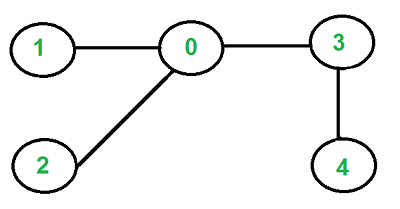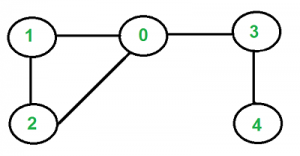Related Articles

# Check if a given graph is tree or not

• Difficulty Level : Medium
• Last Updated : 25 Jun, 2021

Write a function that returns true if a given undirected graph is tree and false otherwise. For example, the following graph is a tree.But the following graph is not a tree.An undirected graph is tree if it has following properties.
1) There is no cycle.
2) The graph is connected.
For an undirected graph we can either use BFS or DFS to detect above two properties.
How to detect cycle in an undirected graph?
We can either use BFS or DFS. For every visited vertex ‘v’, if there is an adjacent ‘u’ such that u is already visited and u is not parent of v, then there is a cycle in graph. If we don’t find such an adjacent for any vertex, we say that there is no cycle (See Detect cycle in an undirected graph for more details).
How to check for connectivity?
Since the graph is undirected, we can start BFS or DFS from any vertex and check if all vertices are reachable or not. If all vertices are reachable, then graph is connected, otherwise not.

## C++

 `// A C++ Program to check whether a graph is tree or not``#include``#include ``#include ``using` `namespace` `std;` `// Class for an undirected graph``class` `Graph``{``    ``int` `V;    ``// No. of vertices``    ``list<``int``> *adj; ``// Pointer to an array for adjacency lists``    ``bool` `isCyclicUtil(``int` `v, ``bool` `visited[], ``int` `parent);``public``:``    ``Graph(``int` `V);   ``// Constructor``    ``void` `addEdge(``int` `v, ``int` `w);   ``// to add an edge to graph``    ``bool` `isTree();   ``// returns true if graph is tree``};` `Graph::Graph(``int` `V)``{``    ``this``->V = V;``    ``adj = ``new` `list<``int``>[V];``}` `void` `Graph::addEdge(``int` `v, ``int` `w)``{``    ``adj[v].push_back(w); ``// Add w to v’s list.``    ``adj[w].push_back(v); ``// Add v to w’s list.``}` `// A recursive function that uses visited[] and parent to``// detect cycle in subgraph reachable from vertex v.``bool` `Graph::isCyclicUtil(``int` `v, ``bool` `visited[], ``int` `parent)``{``    ``// Mark the current node as visited``    ``visited[v] = ``true``;` `    ``// Recur for all the vertices adjacent to this vertex``    ``list<``int``>::iterator i;``    ``for` `(i = adj[v].begin(); i != adj[v].end(); ++i)``    ``{``        ``// If an adjacent is not visited, then recur for``        ``// that adjacent``        ``if` `(!visited[*i])``        ``{``           ``if` `(isCyclicUtil(*i, visited, v))``              ``return` `true``;``        ``}` `        ``// If an adjacent is visited and not parent of current``        ``// vertex, then there is a cycle.``        ``else` `if` `(*i != parent)``           ``return` `true``;``    ``}``    ``return` `false``;``}` `// Returns true if the graph is a tree, else false.``bool` `Graph::isTree()``{``    ``// Mark all the vertices as not visited and not part of``    ``// recursion stack``    ``bool` `*visited = ``new` `bool``[V];``    ``for` `(``int` `i = 0; i < V; i++)``        ``visited[i] = ``false``;` `    ``// The call to isCyclicUtil serves multiple purposes.``    ``// It returns true if graph reachable from vertex 0``    ``// is cyclcic. It also marks all vertices reachable``    ``// from 0.``    ``if` `(isCyclicUtil(0, visited, -1))``             ``return` `false``;` `    ``// If we find a vertex which is not reachable from 0``    ``// (not marked by isCyclicUtil(), then we return false``    ``for` `(``int` `u = 0; u < V; u++)``        ``if` `(!visited[u])``           ``return` `false``;` `    ``return` `true``;``}` `// Driver program to test above functions``int` `main()``{``    ``Graph g1(5);``    ``g1.addEdge(1, 0);``    ``g1.addEdge(0, 2);``    ``g1.addEdge(0, 3);``    ``g1.addEdge(3, 4);``    ``g1.isTree()? cout << ``"Graph is Tree\n"``:``                 ``cout << ``"Graph is not Tree\n"``;` `    ``Graph g2(5);``    ``g2.addEdge(1, 0);``    ``g2.addEdge(0, 2);``    ``g2.addEdge(2, 1);``    ``g2.addEdge(0, 3);``    ``g2.addEdge(3, 4);``    ``g2.isTree()? cout << ``"Graph is Tree\n"``:``                 ``cout << ``"Graph is not Tree\n"``;` `    ``return` `0;``}`

## Java

 `// A Java Program to check whether a graph is tree or not``import` `java.io.*;``import` `java.util.*;` `// This class represents a directed graph using adjacency``// list representation``class` `Graph``{``    ``private` `int` `V; ``// No. of vertices``    ``private` `LinkedList adj[]; ``//Adjacency List` `    ``// Constructor``  ` `    ``@SuppressWarnings``(``"unchecked"``)``    ``Graph(``int` `v)``    ``{``        ``V = v;``        ``adj = ``new` `LinkedList[V];``        ``for` `(``int` `i=``0``; i();``    ``}` `    ``// Function to add an edge into the graph``    ``void` `addEdge(``int` `v,``int` `w)``    ``{``        ``adj[v].add(w);``        ``adj[w].add(v);``    ``}` `    ``// A recursive function that uses visited[] and parent``    ``// to detect cycle in subgraph reachable from vertex v.``    ``boolean` `isCyclicUtil(``int` `v, ``boolean` `visited[], ``int` `parent)``    ``{``        ``// Mark the current node as visited``        ``visited[v] = ``true``;``        ``Integer i;` `        ``// Recur for all the vertices adjacent to this vertex``        ``Iterator it = adj[v].iterator();``        ``while` `(it.hasNext())``        ``{``            ``i = it.next();` `            ``// If an adjacent is not visited, then recur for``            ``// that adjacent``            ``if` `(!visited[i])``            ``{``                ``if` `(isCyclicUtil(i, visited, v))``                    ``return` `true``;``            ``}` `            ``// If an adjacent is visited and not parent of``            ``// current vertex, then there is a cycle.``            ``else` `if` `(i != parent)``            ``return` `true``;``        ``}``        ``return` `false``;``    ``}` `    ``// Returns true if the graph is a tree, else false.``    ``boolean` `isTree()``    ``{``        ``// Mark all the vertices as not visited and not part``        ``// of recursion stack``        ``boolean` `visited[] = ``new` `boolean``[V];``        ``for` `(``int` `i = ``0``; i < V; i++)``            ``visited[i] = ``false``;` `        ``// The call to isCyclicUtil serves multiple purposes``        ``// It returns true if graph reachable from vertex 0``        ``// is cyclcic. It also marks all vertices reachable``        ``// from 0.``        ``if` `(isCyclicUtil(``0``, visited, -``1``))``            ``return` `false``;` `        ``// If we find a vertex which is not reachable from 0``        ``// (not marked by isCyclicUtil(), then we return false``        ``for` `(``int` `u = ``0``; u < V; u++)``            ``if` `(!visited[u])``                ``return` `false``;` `        ``return` `true``;``    ``}` `    ``// Driver method``    ``public` `static` `void` `main(String args[])``    ``{``        ``// Create a graph given in the above diagram``        ``Graph g1 = ``new` `Graph(``5``);``        ``g1.addEdge(``1``, ``0``);``        ``g1.addEdge(``0``, ``2``);``        ``g1.addEdge(``0``, ``3``);``        ``g1.addEdge(``3``, ``4``);``        ``if` `(g1.isTree())``            ``System.out.println(``"Graph is Tree"``);``        ``else``            ``System.out.println(``"Graph is not Tree"``);` `        ``Graph g2 = ``new` `Graph(``5``);``        ``g2.addEdge(``1``, ``0``);``        ``g2.addEdge(``0``, ``2``);``        ``g2.addEdge(``2``, ``1``);``        ``g2.addEdge(``0``, ``3``);``        ``g2.addEdge(``3``, ``4``);` `        ``if` `(g2.isTree())``            ``System.out.println(``"Graph is Tree"``);``        ``else``            ``System.out.println(``"Graph is not Tree"``);` `    ``}``}``// This code is contributed by Aakash Hasija`

## Python

 `# Python Program to check whether``# a graph is tree or not` `from` `collections ``import` `defaultdict` `class` `Graph():` `    ``def` `__init__(``self``, V):``        ``self``.V ``=` `V``        ``self``.graph  ``=` `defaultdict(``list``)` `    ``def` `addEdge(``self``, v, w):``        ``# Add w to v ist.``        ``self``.graph[v].append(w)``        ``# Add v to w list.``        ``self``.graph[w].append(v)` `    ``# A recursive function that uses visited[]``    ``# and parent to detect cycle in subgraph``    ``# reachable from vertex v.``    ``def` `isCyclicUtil(``self``, v, visited, parent):` `        ``# Mark current node as visited``        ``visited[v] ``=` `True` `        ``# Recur for all the vertices adjacent``        ``# for this vertex``        ``for` `i ``in` `self``.graph[v]:``            ``# If an adjacent is not visited,``            ``# then recur for that adjacent``            ``if` `visited[i] ``=``=` `False``:``                ``if` `self``.isCyclicUtil(i, visited, v) ``=``=` `True``:``                    ``return` `True` `            ``# If an adjacent is visited and not``            ``# parent of current vertex, then there``            ``# is a cycle.``            ``elif` `i !``=` `parent:``                ``return` `True` `        ``return` `False` `    ``# Returns true if the graph is a tree,``    ``# else false.``    ``def` `isTree(``self``):``        ``# Mark all the vertices as not visited``        ``# and not part of recursion stack``        ``visited ``=` `[``False``] ``*` `self``.V` `        ``# The call to isCyclicUtil serves multiple``        ``# purposes. It returns true if graph reachable``        ``# from vertex 0 is cyclcic. It also marks``        ``# all vertices reachable from 0.``        ``if` `self``.isCyclicUtil(``0``, visited, ``-``1``) ``=``=` `True``:``            ``return` `False` `        ``# If we find a vertex which is not reachable``        ``# from 0 (not marked by isCyclicUtil(),``        ``# then we return false``        ``for` `i ``in` `range``(``self``.V):``            ``if` `visited[i] ``=``=` `False``:``                ``return` `False` `        ``return` `True` `# Driver program to test above functions``g1 ``=` `Graph(``5``)``g1.addEdge(``1``, ``0``)``g1.addEdge(``0``, ``2``)``g1.addEdge(``0``, ``3``)``g1.addEdge(``3``, ``4``)``print` `"Graph is a Tree"` `if` `g1.isTree() ``=``=` `True` `\``                          ``else` `"Graph is a not a Tree"` `g2 ``=` `Graph(``5``)``g2.addEdge(``1``, ``0``)``g2.addEdge(``0``, ``2``)``g2.addEdge(``2``, ``1``)``g2.addEdge(``0``, ``3``)``g2.addEdge(``3``, ``4``)``print` `"Graph is a Tree"` `if` `g2.isTree() ``=``=` `True` `\``                          ``else` `"Graph is a not a Tree"``                          ` `# This code is contributed by Divyanshu Mehta     `

## C#

 `// A C# Program to check whether``// a graph is tree or not``using` `System;``using` `System.Collections.Generic;` `// This class represents a directed graph``// using adjacency list representation``class` `Graph``{``    ``private` `int` `V; ``// No. of vertices``    ``private` `List<``int``> []adj; ``// Adjacency List` `    ``// Constructor``    ``Graph(``int` `v)``    ``{``        ``V = v;``        ``adj = ``new` `List<``int``>[v];``        ``for` `(``int` `i = 0; i < v; ++i)``            ``adj[i] = ``new` `List<``int``>();``    ``}` `    ``// Function to add an edge``    ``// into the graph``    ``void` `addEdge(``int` `v, ``int` `w)``    ``{``        ``adj[v].Add(w);``        ``adj[w].Add(v);``    ``}` `    ``// A recursive function that uses visited[]``    ``// and parent to detect cycle in subgraph``    ``// reachable from vertex v.``    ``Boolean isCyclicUtil(``int` `v, Boolean []visited,``                         ``int` `parent)``    ``{``        ``// Mark the current node as visited``        ``visited[v] = ``true``;``        ``int` `i;` `        ``// Recur for all the vertices``        ``// adjacent to this vertex``        ``foreach``(``int` `it ``in` `adj[v])``        ``{``            ``i = it;` `            ``// If an adjacent is not visited,``            ``// then recur for that adjacent``            ``if` `(!visited[i])``            ``{``                ``if` `(isCyclicUtil(i, visited, v))``                    ``return` `true``;``            ``}` `            ``// If an adjacent is visited and``            ``// not parent of current vertex,``            ``// then there is a cycle.``            ``else` `if` `(i != parent)``            ``return` `true``;``        ``}``        ``return` `false``;``    ``}` `    ``// Returns true if the graph``    ``// is a tree, else false.``    ``Boolean isTree()``    ``{``        ``// Mark all the vertices as not visited``        ``// and not part of recursion stack``        ``Boolean []visited = ``new` `Boolean[V];``        ``for` `(``int` `i = 0; i < V; i++)``            ``visited[i] = ``false``;` `        ``// The call to isCyclicUtil serves``        ``// multiple purposes. It returns true if``        ``// graph reachable from vertex 0 is cyclcic.``        ``// It also marks all vertices reachable from 0.``        ``if` `(isCyclicUtil(0, visited, -1))``            ``return` `false``;` `        ``// If we find a vertex which is not reachable``        ``// from 0 (not marked by isCyclicUtil(),``        ``// then we return false``        ``for` `(``int` `u = 0; u < V; u++)``            ``if` `(!visited[u])``                ``return` `false``;` `        ``return` `true``;``    ``}` `    ``// Driver Code``    ``public` `static` `void` `Main(String []args)``    ``{``        ``// Create a graph given``        ``// in the above diagram``        ``Graph g1 = ``new` `Graph(5);``        ``g1.addEdge(1, 0);``        ``g1.addEdge(0, 2);``        ``g1.addEdge(0, 3);``        ``g1.addEdge(3, 4);``        ``if` `(g1.isTree())``            ``Console.WriteLine(``"Graph is Tree"``);``        ``else``            ``Console.WriteLine(``"Graph is not Tree"``);` `        ``Graph g2 = ``new` `Graph(5);``        ``g2.addEdge(1, 0);``        ``g2.addEdge(0, 2);``        ``g2.addEdge(2, 1);``        ``g2.addEdge(0, 3);``        ``g2.addEdge(3, 4);` `        ``if` `(g2.isTree())``            ``Console.WriteLine(``"Graph is Tree"``);``        ``else``            ``Console.WriteLine(``"Graph is not Tree"``);` `    ``}``}` `// This code is contributed by Rajput-Ji`

## Javascript

 ``

Output:

```Graph is Tree
Graph is not Tree```

Thanks to Vinit Verma for suggesting this problem and initial solution. Please write comments if you find anything incorrect, or you want to share more information about the topic discussed above

Attention reader! Don’t stop learning now. Get hold of all the important DSA concepts with the DSA Self Paced Course at a student-friendly price and become industry ready.  To complete your preparation from learning a language to DS Algo and many more,  please refer Complete Interview Preparation Course.

In case you wish to attend live classes with experts, please refer DSA Live Classes for Working Professionals and Competitive Programming Live for Students.

My Personal Notes arrow_drop_up